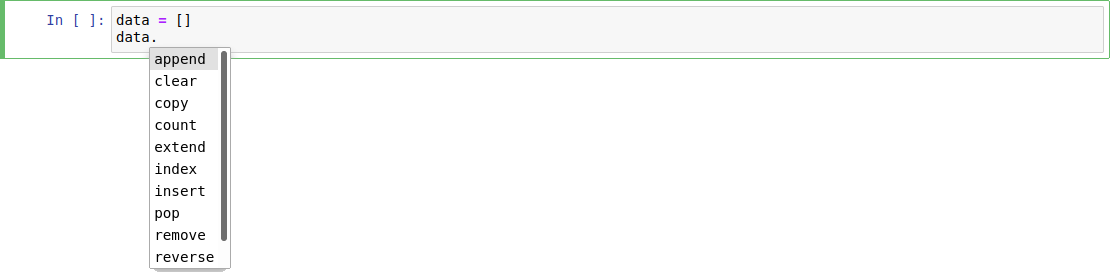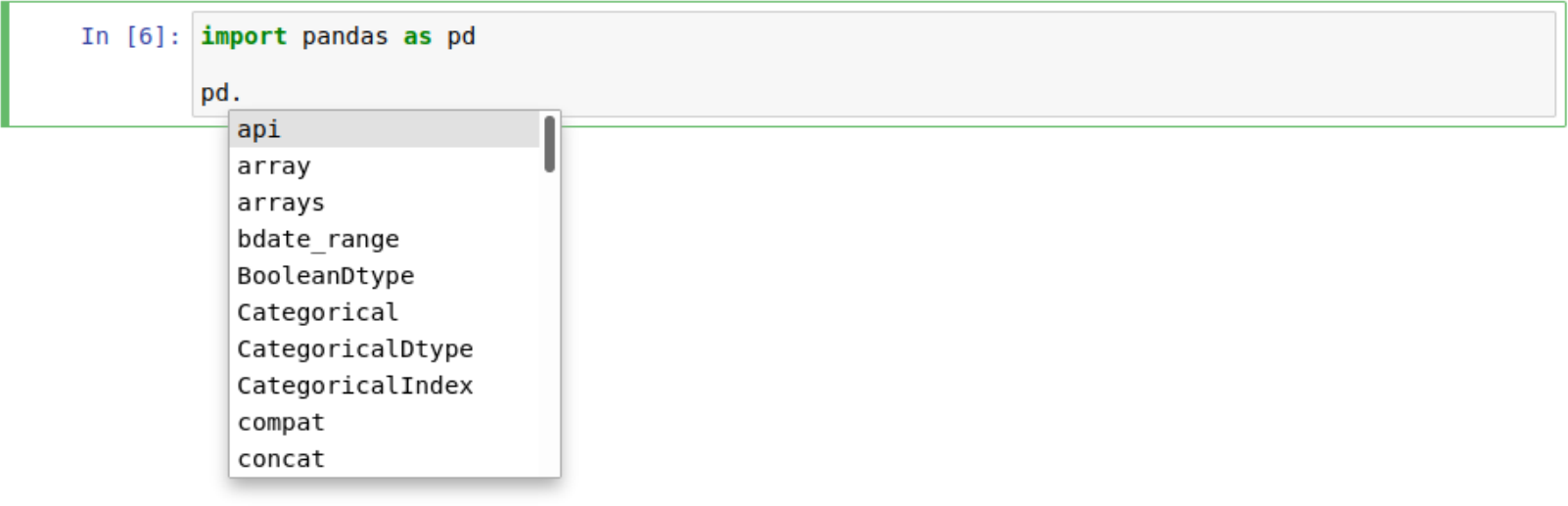# IPython examples#

## Running Python code#

### Show Python version#

:

import sys

sys.version_info

:

sys.version_info(major=3, minor=11, micro=4, releaselevel='final', serial=0)


### Show versions of Python packages#

Most Python packages provide a __version__ method for this:

:

import pandas as pd

pd.__version__

:

'2.0.3'


Alternatively, you can use version from importlib_metadata:

:

from importlib_metadata import version

print(version("pandas"))

2.0.3


### Information about the host operating system and the versions of installed Python packages#

:

pd.show_versions()

/Users/veit/.local/share/virtualenvs/python-311-6zxVKbDJ/lib/python3.11/site-packages/_distutils_hack/__init__.py:33: UserWarning: Setuptools is replacing distutils.
warnings.warn("Setuptools is replacing distutils.")


INSTALLED VERSIONS
------------------
commit           : 0f437949513225922d851e9581723d82120684a6
python           : 3.11.4.final.0
python-bits      : 64
OS               : Darwin
OS-release       : 22.5.0
Version          : Darwin Kernel Version 22.5.0: Thu Jun  8 22:22:23 PDT 2023; root:xnu-8796.121.3~7/RELEASE_ARM64_T6020
machine          : arm64
processor        : arm
byteorder        : little
LC_ALL           : None
LANG             : de_DE.UTF-8
LOCALE           : de_DE.UTF-8

pandas           : 2.0.3
numpy            : 1.23.5
pytz             : 2023.3
dateutil         : 2.8.2
setuptools       : 68.0.0
pip              : 23.1.2
Cython           : None
pytest           : 7.4.0
hypothesis       : 6.81.1
sphinx           : 7.0.1
blosc            : None
feather          : None
xlsxwriter       : None
lxml.etree       : 4.9.3
html5lib         : None
pymysql          : None
psycopg2         : None
jinja2           : 3.1.2
IPython          : 8.14.0
bs4              : 4.12.2
bottleneck       : None
brotli           : None
fastparquet      : 2023.7.0
fsspec           : 2023.6.0
gcsfs            : 2023.6.0
matplotlib       : 3.7.2
numba            : 0.57.1
numexpr          : None
odfpy            : None
openpyxl         : None
pandas_gbq       : None
pyarrow          : 12.0.1
pyxlsb           : None
s3fs             : 2023.6.0
scipy            : 1.11.1
snappy           : None
sqlalchemy       : None
tables           : None
tabulate         : 0.9.0
xarray           : 2023.6.0
xlrd             : None
zstandard        : None
tzdata           : 2023.3
qtpy             : None
pyqt5            : None


### Only use Python versions ≥ 3.8#

:

import sys

assert sys.version_info[:2] >= (3, 8)


## Shell commands#

:

!python3 -V

Python 3.11.4

:

!python3 -m pip --version

pip 23.1.2 from /Users/veit/.local/share/virtualenvs/python-311-6zxVKbDJ/lib/python3.11/site-packages/pip (python 3.11)


## Tab completion#

… for objects with methods and attributes:… and also for modules:Note:

As you may have noticed in surprise, the __version__ method used above is not offered in the selection. IPython initially hides these private methods and attributes that begin with underscores. However, they can also be completed with a tabulator if you first enter an underscore. Alternatively, you can change this setting in the IPython configuration.

… for almost everything:## Displaying information about an object#

With a question mark (?) you can display information about an object if, for example, there is a method multiply with the following docstring:

:

import numpy as np

:

np.mean?

Signature:
np.mean(
a,
axis=None,
dtype=None,
out=None,
keepdims=<no value>,
*,
where=<no value>,
)
Docstring:
Compute the arithmetic mean along the specified axis.

Returns the average of the array elements.  The average is taken over
the flattened array by default, otherwise over the specified axis.
float64 intermediate and return values are used for integer inputs.

Parameters
----------
a : array_like
Array containing numbers whose mean is desired. If a is not an
array, a conversion is attempted.
axis : None or int or tuple of ints, optional
Axis or axes along which the means are computed. The default is to
compute the mean of the flattened array.

If this is a tuple of ints, a mean is performed over multiple axes,
instead of a single axis or all the axes as before.
dtype : data-type, optional
Type to use in computing the mean.  For integer inputs, the default
is float64; for floating point inputs, it is the same as the
input dtype.
out : ndarray, optional
Alternate output array in which to place the result.  The default
is None; if provided, it must have the same shape as the
expected output, but the type will be cast if necessary.
See :ref:ufuncs-output-type for more details.

keepdims : bool, optional
If this is set to True, the axes which are reduced are left
in the result as dimensions with size one. With this option,
the result will broadcast correctly against the input array.

If the default value is passed, then keepdims will not be
passed through to the mean method of sub-classes of
ndarray, however any non-default value will be.  If the
sub-class' method does not implement keepdims any
exceptions will be raised.

where : array_like of bool, optional
Elements to include in the mean. See ~numpy.ufunc.reduce for details.

Returns
-------
m : ndarray, see dtype parameter above
If out=None, returns a new array containing the mean values,
otherwise a reference to the output array is returned.

--------
average : Weighted average
std, var, nanmean, nanstd, nanvar

Notes
-----
The arithmetic mean is the sum of the elements along the axis divided
by the number of elements.

Note that for floating-point input, the mean is computed using the
same precision the input has.  Depending on the input data, this can
cause the results to be inaccurate, especially for float32 (see
example below).  Specifying a higher-precision accumulator using the
dtype keyword can alleviate this issue.

By default, float16 results are computed using float32 intermediates
for extra precision.

Examples
--------
>>> a = np.array([[1, 2], [3, 4]])
>>> np.mean(a)
2.5
>>> np.mean(a, axis=0)
array([2., 3.])
>>> np.mean(a, axis=1)
array([1.5, 3.5])

In single precision, mean can be inaccurate:

>>> a = np.zeros((2, 512*512), dtype=np.float32)
>>> a[0, :] = 1.0
>>> a[1, :] = 0.1
>>> np.mean(a)
0.54999924

Computing the mean in float64 is more accurate:

>>> np.mean(a, dtype=np.float64)
0.55000000074505806 # may vary

Specifying a where argument:
>>> a = np.array([[5, 9, 13], [14, 10, 12], [11, 15, 19]])
>>> np.mean(a)
12.0
>>> np.mean(a, where=[[True], [False], [False]])
9.0
File:      ~/spack/var/spack/environments/python-38/.spack-env/view/lib/python3.8/site-packages/numpy/core/fromnumeric.py
Type:      function


By using ?? the source code of the function is also displayed, if this is possible:

:

np.mean??

Signature:
np.mean(
a,
axis=None,
dtype=None,
out=None,
keepdims=<no value>,
*,
where=<no value>,
)
Source:
@array_function_dispatch(_mean_dispatcher)
def mean(a, axis=None, dtype=None, out=None, keepdims=np._NoValue, *,
where=np._NoValue):
"""
Compute the arithmetic mean along the specified axis.

Returns the average of the array elements.  The average is taken over
the flattened array by default, otherwise over the specified axis.
float64 intermediate and return values are used for integer inputs.

Parameters
----------
a : array_like
Array containing numbers whose mean is desired. If a is not an
array, a conversion is attempted.
axis : None or int or tuple of ints, optional
Axis or axes along which the means are computed. The default is to
compute the mean of the flattened array.

If this is a tuple of ints, a mean is performed over multiple axes,
instead of a single axis or all the axes as before.
dtype : data-type, optional
Type to use in computing the mean.  For integer inputs, the default
is float64; for floating point inputs, it is the same as the
input dtype.
out : ndarray, optional
Alternate output array in which to place the result.  The default
is None; if provided, it must have the same shape as the
expected output, but the type will be cast if necessary.
See :ref:ufuncs-output-type for more details.

keepdims : bool, optional
If this is set to True, the axes which are reduced are left
in the result as dimensions with size one. With this option,
the result will broadcast correctly against the input array.

If the default value is passed, then keepdims will not be
passed through to the mean method of sub-classes of
ndarray, however any non-default value will be.  If the
sub-class' method does not implement keepdims any
exceptions will be raised.

where : array_like of bool, optional
Elements to include in the mean. See ~numpy.ufunc.reduce for details.

Returns
-------
m : ndarray, see dtype parameter above
If out=None, returns a new array containing the mean values,
otherwise a reference to the output array is returned.

--------
average : Weighted average
std, var, nanmean, nanstd, nanvar

Notes
-----
The arithmetic mean is the sum of the elements along the axis divided
by the number of elements.

Note that for floating-point input, the mean is computed using the
same precision the input has.  Depending on the input data, this can
cause the results to be inaccurate, especially for float32 (see
example below).  Specifying a higher-precision accumulator using the
dtype keyword can alleviate this issue.

By default, float16 results are computed using float32 intermediates
for extra precision.

Examples
--------
>>> a = np.array([[1, 2], [3, 4]])
>>> np.mean(a)
2.5
>>> np.mean(a, axis=0)
array([2., 3.])
>>> np.mean(a, axis=1)
array([1.5, 3.5])

In single precision, mean can be inaccurate:

>>> a = np.zeros((2, 512*512), dtype=np.float32)
>>> a[0, :] = 1.0
>>> a[1, :] = 0.1
>>> np.mean(a)
0.54999924

Computing the mean in float64 is more accurate:

>>> np.mean(a, dtype=np.float64)
0.55000000074505806 # may vary

Specifying a where argument:
>>> a = np.array([[5, 9, 13], [14, 10, 12], [11, 15, 19]])
>>> np.mean(a)
12.0
>>> np.mean(a, where=[[True], [False], [False]])
9.0

"""
kwargs = {}
if keepdims is not np._NoValue:
kwargs['keepdims'] = keepdims
if where is not np._NoValue:
kwargs['where'] = where
if type(a) is not mu.ndarray:
try:
mean = a.mean
except AttributeError:
pass
else:
return mean(axis=axis, dtype=dtype, out=out, **kwargs)

return _methods._mean(a, axis=axis, dtype=dtype,
out=out, **kwargs)
File:      ~/spack/var/spack/environments/python-38/.spack-env/view/lib/python3.8/site-packages/numpy/core/fromnumeric.py
Type:      function


? can also be used to search in the IPython namespace. In doing so, a series of characters can be represented with the wildcard (*). For example, to get a list of all functions in the top-level NumPy namespace that contain mean:

:

np.*mean*?

np.mean
np.nanmean